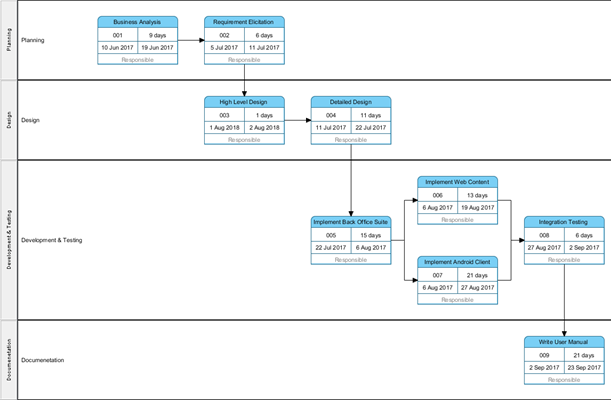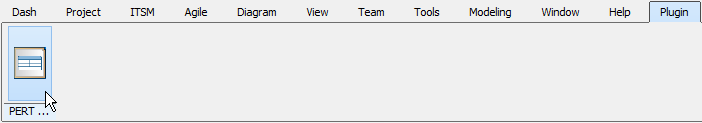# Create a PERT Diagram Using Open API

Program Evaluation Review Technique (PERT) is a technique to schedule, organize and coordinate tasks of a project. This article will demonstrate how to create a PERT Chart using Open API.The PERT Chart will be created by the plugin

## Create Blank PERT Diagram

A blank PERT Diagram is first created using the Diagrammanager.createDiagram() method.

```// Create blank diagram
DiagramManager diagramManager = ApplicationManager.instance().getDiagramManager();
IDiagramUIModel pert = (IDiagramUIModel) diagramManager.createDiagram(IDiagramTypeConstants.DIAGRAM_TYPE_PERT_CHART);
pert.setName("Sample PERT Chart");```

## Create Lanes

Once the diagram is created, we are ready to create some lanes from IModelElementFactory. Here is an example to create a lane.

```// Create Planning Lane
IPERTLane planning = IModelElementFactory.instance().createPERTLane();
planning.setName("Planning");
//display lane on the diagram
IPERTLaneUIModel shapePlanning = (IPERTLaneUIModel) diagramManager.createDiagramElement(pert, planning);
shapePlanning.setBounds(0, 0, 1150, 150);
shapePlanning.resetCaption();
shapePlanning.setRequestResetCaption(true);```

A task can be create when the belonging lane is created. In this example, every tasks is a child of its belonging lane, so instead create from IModelElementnFactory, tasks will be created using the method IPERTLane.createPERTTask().

```// Create Tasks on the Planning Lane
//The task is a child of the Planning Lane
//the method getDate() is the method created below
busAna.setStartDate(getDate(2017, 6, 10));
busAna.setFinishDate(getDate(2017, 6, 19));
//display the task on the diagram
shapeBusAna.setBounds(150, 30, 150, 90);
shapeBusAna.resetCaption();```

For convenient, a private method getDate(int year, int month, int date) is created in the class for multiple uses:

```//this is the private method used in the methods IPERTTask.setStartDate and IPERTTask.setFinishDate
private String getDate(int year, int month, int date) {
Calendar calendar = Calendar.getInstance();
calendar.set(Calendar.YEAR, year);
//The month starts form 0 for January to 11 for December
calendar.set(Calendar.MONTH, month - 1);
calendar.set(Calendar.DATE, date);
calendar.set(Calendar.HOUR_OF_DAY, 0);
calendar.set(Calendar.MINUTE, 0);
calendar.set(Calendar.SECOND, 0);
calendar.set(Calendar.MILLISECOND, 0);
long longDate = calendar.getTimeInMillis();
return String.valueOf(longDate);
}```

## Create Dependencies

When all the tasks are created, we can start creating dependencies. You can create a dependencies using IModelElementnFactory.createConnector().

```//Create Dependencies
IPERTDependency busAnaToReqEli = IModelElementFactory.instance().createPERTDependency();
busAnaToReqEli.setFrom(busAna);
busAnaToReqEli.setTo(reqEli);
//Display the dependency on the diagram
diagramManager.createConnector(pert, busAnaToReqEli, shapeBusAna, shapeReqEli, new Point[] {new Point(300,75), new Point (350,75)});```

## Show Up Diagram

Finally, show up the diagram.

```// show up diagram
diagramManager.openDiagram(pert);```

## Sample Plugin

The sample plugin demonstrate how to create PERT Chart using Open API. After you deploy the plugin into Visual Paradigm you can then click the plugin button in the application toolbar to trigger it.